# Phase (waves)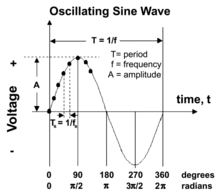The relationship between period, frequency, and amplitude for a sine wave is illustrated in this image

Phase is the position of a point in time (an instant) on a waveform cycle. A complete cycle is defined as the interval required for the waveform to return to its arbitrary initial value. The graphic to the right shows how one cycle constitutes 360° of phase. The graphic also shows how phase is sometimes expressed in radians, where one radian of phase equals approximately 57.3°.

Phase can also be an expression of relative displacement between two corresponding features (for example, peaks or zero crossings) of two waveforms having the same frequency.

In sinusoidal functions or in waves "phase" has two different, but closely related, meanings. One is the initial angle of a sinusoidal function at its origin and is sometimes called phase offset or phase difference. Another usage is the fraction of the wave cycle that has elapsed relative to the origin.

## Phase shift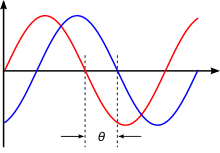Illustration of phase shift. The horizontal axis represents an angle (phase) that is increasing with time.

Phase shift is any change that occurs in the phase of one quantity, or in the phase difference between two or more quantities.

This symbol:is sometimes referred to as a phase shift or phase offset, because it represents a "shift" from zero phase.

For infinitely long sinusoids, a change inis the same as a shift in time, such as a time delay. Ifis delayed (time-shifted) byof its cycle, it becomes:whose "phase" is now. It has been shifted byradians.

## Phase difference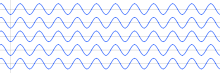In-phase waves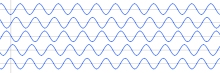Out-of-phase waves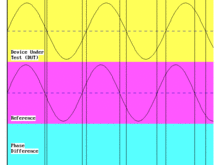Representation of phase comparison.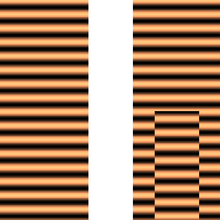Left: the real part of a plane wave moving from top to bottom. Right: the same wave after a central section underwent a phase shift, for example, by passing through a glass of different thickness than the other parts.

Phase difference is the difference, expressed in degrees or time, between two waves having the same frequency and referenced to the same point in time. Two oscillators that have the same frequency and no phase difference are said to be in phase. Two oscillators that have the same frequency and different phases have a phase difference, and the oscillators are said to be out of phase with each other.

The amount by which such oscillators are out of phase with each other can be expressed in degrees from 0° to 360°, or in radians from 0 to 2π. If the phase difference is 180 degrees (π radians), then the two oscillators are said to be in antiphase. If two interacting waves meet at a point where they are in antiphase, then destructive interference will occur. It is common for waves of electromagnetic (light, RF), acoustic (sound) or other energy to become superposed in their transmission medium. When that happens, the phase difference determines whether they reinforce or weaken each other. Complete cancellation is possible for waves with equal amplitudes.

Time is sometimes used (instead of angle) to express position within the cycle of an oscillation. A phase difference is analogous to two athletes running around a race track at the same speed and direction but starting at different positions on the track. They pass a point at different instants in time. But the time difference (phase difference) between them is a constant - same for every pass since they are at the same speed and in the same direction. If they were at different speeds (different frequencies), the phase difference is undefined and would only reflect different starting positions. Technically, phase difference between two entities at various frequencies is undefined and does not exist.

• Time zones are also analogous to phase differences.

A real-world example of a sonic phase difference occurs in the warble of a Native American flute. The amplitude of different harmonic components of same long-held note on the flute come into dominance at different points in the phase cycle. The phase difference between the different harmonics can be observed on a spectrogram of the sound of a warbling flute.

### Phase comparison

Phase comparison is a comparison of the phase of two waveforms, usually of the same nominal frequency. In time and frequency, the purpose of a phase comparison is generally to determine the frequency offset (difference between wave cycles) with respect to a reference.

A phase comparison can be made by connecting two signals to a two-channel oscilloscope. The oscilloscope will display two sine waves, as shown in the graphic to the right. In the adjacent image, the top sine wave is the test frequency, and the bottom sine wave represents a signal from the reference.

If the two frequencies were exactly the same, their phase relationship would not change and both would appear to be stationary on the oscilloscope display. Since the two frequencies are not exactly the same, the reference appears to be stationary and the test signal moves. By measuring the rate of motion of the test signal the offset between frequencies can be determined.

Vertical lines have been drawn through the points where each sine wave passes through zero. The bottom of the figure shows bars whose width represents the phase difference between the signals. In this case the phase difference is increasing, indicating that the test signal is lower in frequency than the reference.

## Formula for phase of an oscillation or a wave

The phase of an oscillation or wave refers to a sinusoidal function such as the following:where,, andare constant parameters called the amplitude, frequency, and phase of the sinusoid. These functions are periodic with period, and they are identical except for a displacement ofalong theaxis. The term phase can refer to several different things:

• It can refer to a specified reference, such as, in which case we would say the phase ofis, and the phase ofis.
• It can refer to, in which case we would sayandhave the same phase but are relative to their own specific references.
• In the context of communication waveforms, the time-variant angle, or its principal value, is referred to as instantaneous phase, often just phase.Wikimedia Commons has media related to Phase (waves).